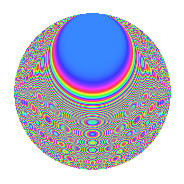# Properties

 Label 1512.2.btLevel 1512 Weight 2 Character orbit bt Rep. character $$\chi_{1512}(611,\cdot)$$ Character field $$\Q(\zeta_{6})$$ Dimension 184 Sturm bound 576

# Related objects

## Defining parameters

 Level: $$N$$ = $$1512 = 2^{3} \cdot 3^{3} \cdot 7$$ Weight: $$k$$ = $$2$$ Character orbit: $$[\chi]$$ = 1512.bt (of order $$6$$ and degree $$2$$) Character conductor: $$\operatorname{cond}(\chi)$$ = $$504$$ Character field: $$\Q(\zeta_{6})$$ Sturm bound: $$576$$

## Dimensions

The following table gives the dimensions of various subspaces of $$M_{2}(1512, [\chi])$$.

Total New Old
Modular forms 600 200 400
Cusp forms 552 184 368
Eisenstein series 48 16 32

## Trace form

 $$184q - 2q^{4} + O(q^{10})$$ $$184q - 2q^{4} - 6q^{10} + 6q^{11} - 12q^{14} - 2q^{16} - 4q^{19} + 6q^{20} + 2q^{22} - 74q^{25} + 6q^{26} - 4q^{34} - 30q^{35} - 39q^{38} + 6q^{40} + 12q^{41} - 4q^{43} - 9q^{44} - 6q^{46} - 2q^{49} + 21q^{50} + 9q^{52} + 24q^{56} - 3q^{58} - 8q^{64} - 4q^{67} + 42q^{68} - 3q^{70} - 4q^{73} - 27q^{74} + 2q^{76} - 87q^{80} - 4q^{82} + 72q^{83} + 27q^{86} - 7q^{88} + 24q^{89} - 36q^{91} + 36q^{92} - 18q^{94} - 4q^{97} - 57q^{98} + O(q^{100})$$

## Decomposition of $$S_{2}^{\mathrm{new}}(1512, [\chi])$$ into newform subspaces

The newforms in this space have not yet been added to the LMFDB.

## Decomposition of $$S_{2}^{\mathrm{old}}(1512, [\chi])$$ into lower level spaces

$$S_{2}^{\mathrm{old}}(1512, [\chi]) \cong$$ $$S_{2}^{\mathrm{new}}(504, [\chi])$$$$^{\oplus 2}$$

## Hecke Characteristic Polynomials

There are no characteristic polynomials of Hecke operators in the database